# Difference between revisions of "Velocity Verlet algorithm"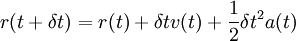$r(t + \delta t) = r (t) + \delta t v(t) + \frac{1}{2} \delta t^2 a(t)$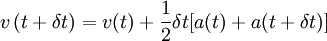$v \left(t+ \delta t\right) = v(t) + \frac{1}{2} \delta t [ a(t) + a(t+\delta t)]$
where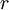$r$ is the position,$v$ is the velocity,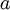$a$ is the acceleration and$t$ is the time.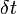$\delta t$ is known as the time step.